# Selina Solutions Concise Mathematics Class 6 Chapter 29 The Circle Exercise 29(A)

Selina Solutions Concise Mathematics Class 6 Chapter 29 The Circle Exercise 29(A) helps students to understand the basic concepts, which are vital from the exam point of view. Parts of Circle are the major topics which are covered here. The solutions are provided in a descriptive manner to enhance conceptual understanding among the students. Thorough practice of these solutions helps them to solve difficult problems with ease, along with securing high marks in the exam. For increasing problem solving skills in a short duration, students can follow Selina Solutions Concise Mathematics Class 6 Chapter 29 The Circle Exercise 29(A) free PDF, from the links mentioned here.

## Selina Solutions Concise Mathematics Class 6 Chapter 29 The Circle Exercise 29(A) Download PDF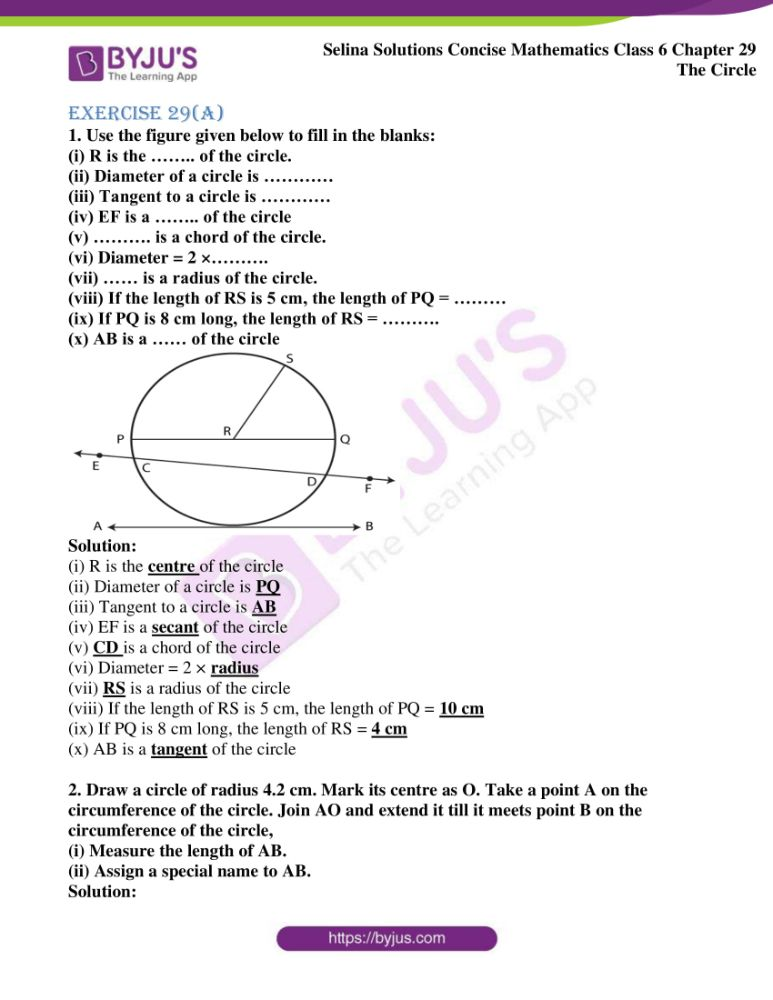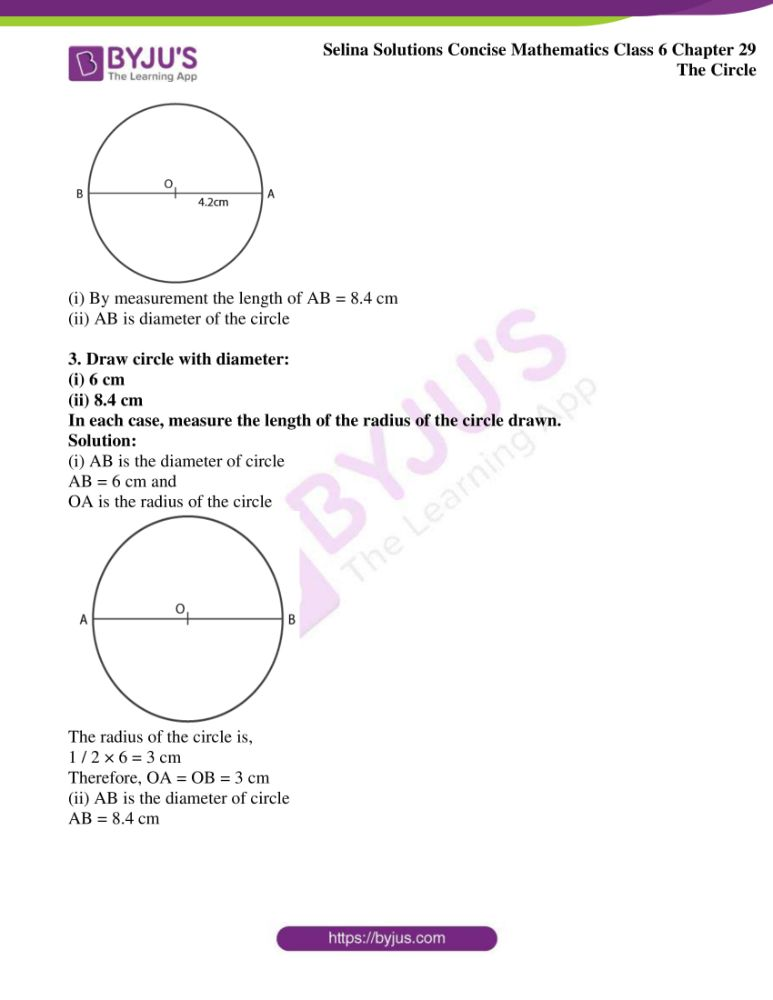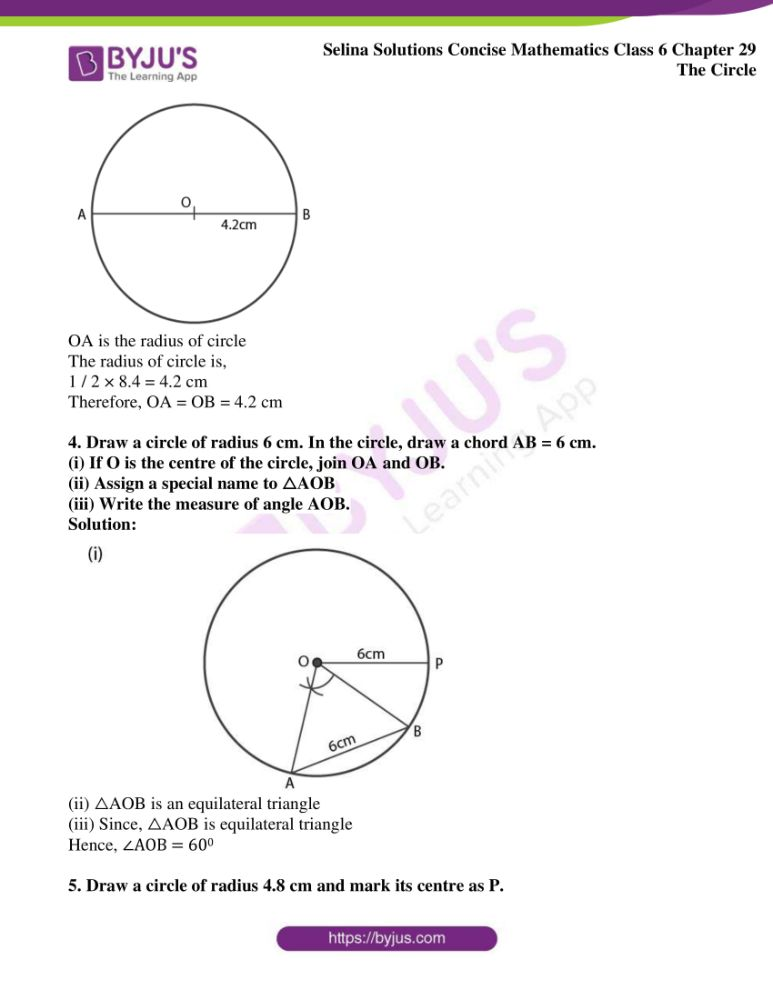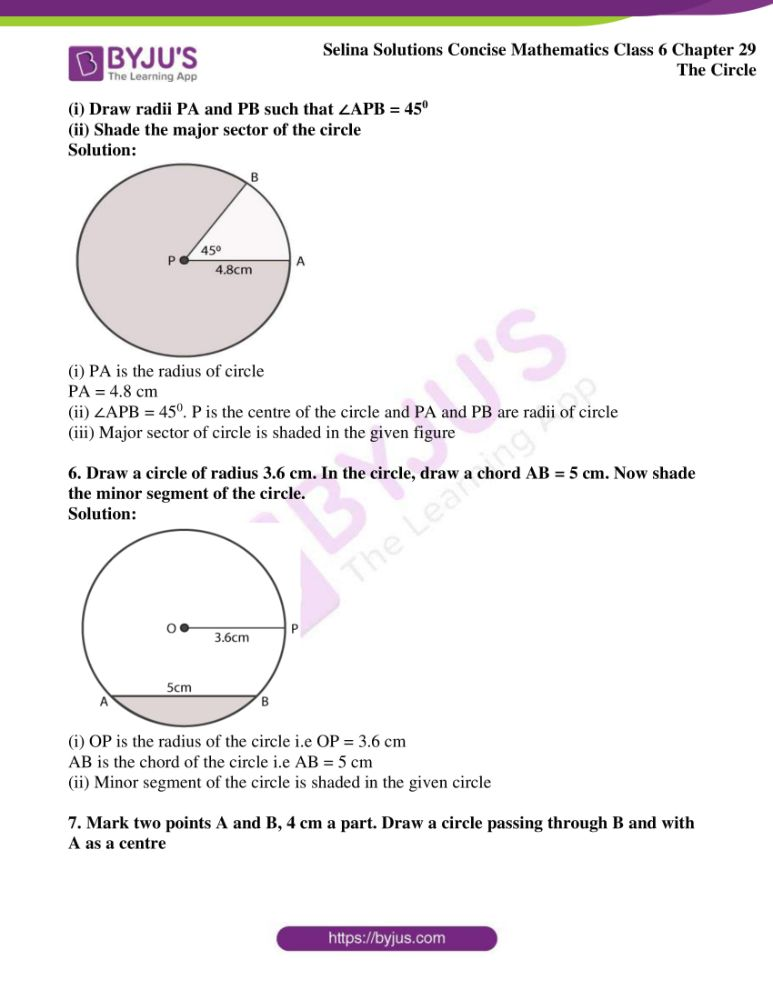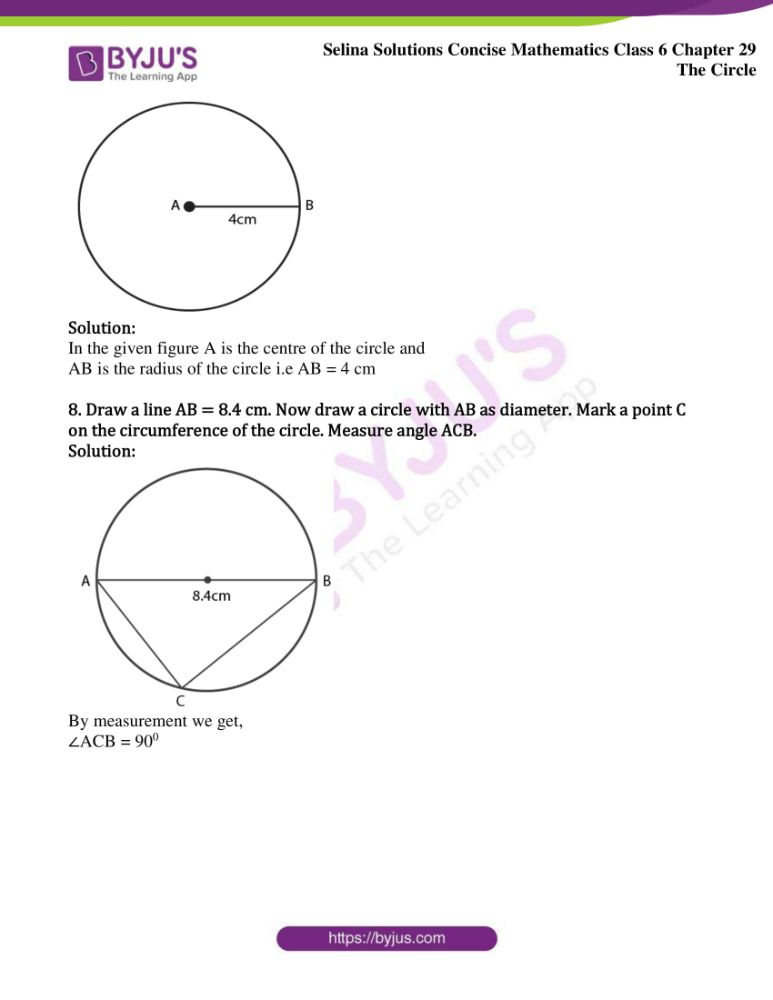### Access Selina Solutions Concise Mathematics Class 6 Chapter 29 The Circle Exercise 29(A)

Exercise 29(A)

1. Use the figure given below to fill in the blanks:

(i) R is the …….. of the circle.

(ii) Diameter of a circle is …………

(iii) Tangent to a circle is …………

(iv) EF is a …….. of the circle

(v) ………. is a chord of the circle.

(vi) Diameter = 2 ×……….

(vii) …… is a radius of the circle.

(viii) If the length of RS is 5 cm, the length of PQ = ………

(ix) If PQ is 8 cm long, the length of RS = ……….

(x) AB is a …… of the circle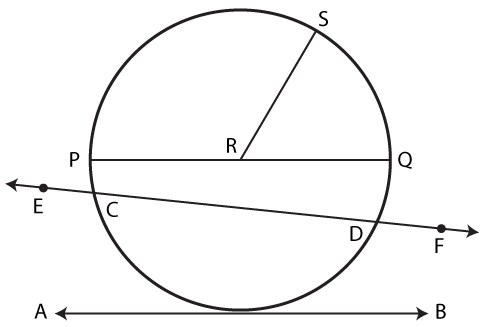Solution:

(i) R is the centre of the circle

(ii) Diameter of a circle is PQ

(iii) Tangent to a circle is AB

(iv) EF is a secant of the circle

(v) CD is a chord of the circle

(vi) Diameter = 2 × radius

(vii) RS is a radius of the circle

(viii) If the length of RS is 5 cm, the length of PQ = 10 cm

(ix) If PQ is 8 cm long, the length of RS = 4 cm

(x) AB is a tangent of the circle

2. Draw a circle of radius 4.2 cm. Mark its centre as O. Take a point A on the circumference of the circle. Join AO and extend it till it meets point B on the circumference of the circle,

(i) Measure the length of AB.

(ii) Assign a special name to AB.

Solution: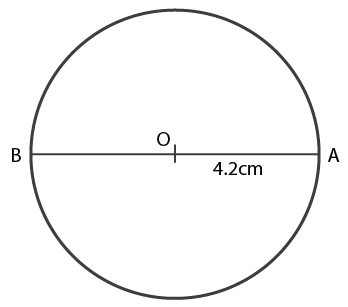(i) By measurement the length of AB = 8.4 cm

(ii) AB is diameter of the circle

3. Draw circle with diameter:

(i) 6 cm

(ii) 8.4 cm

In each case, measure the length of the radius of the circle drawn.

Solution:

(i) AB is the diameter of circle

AB = 6 cm and

OA is the radius of the circle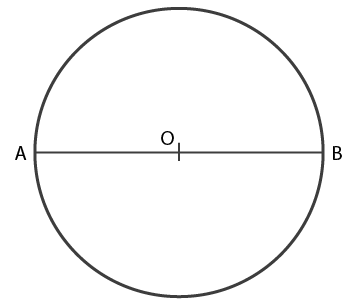The radius of the circle is,

1 / 2 × 6 = 3 cm

Therefore, OA = OB = 3 cm

(ii) AB is the diameter of circle

AB = 8.4 cm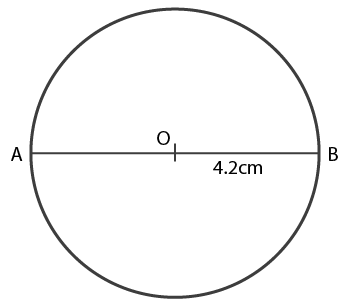OA is the radius of circle

1 / 2 × 8.4 = 4.2 cm

Therefore, OA = OB = 4.2 cm

4. Draw a circle of radius 6 cm. In the circle, draw a chord AB = 6 cm.

(i) If O is the centre of the circle, join OA and OB.

(ii) Assign a special name to △AOB

(iii) Write the measure of angle AOB.

Solution: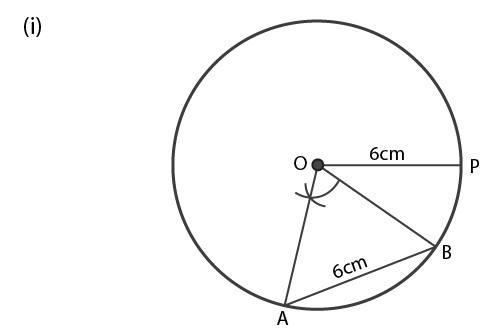(ii) △AOB is an equilateral triangle

(iii) Since, △AOB is equilateral triangle

Hence, ∠AOB = 600

5. Draw a circle of radius 4.8 cm and mark its centre as P.

(i) Draw radii PA and PB such that ∠APB = 450

(ii) Shade the major sector of the circle

Solution: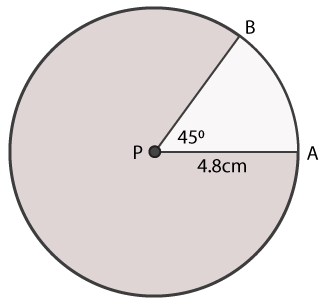(i) PA is the radius of circle

PA = 4.8 cm

(ii) ∠APB = 450. P is the centre of the circle and PA and PB are radii of circle

(iii) Major sector of circle is shaded in the given figure

6. Draw a circle of radius 3.6 cm. In the circle, draw a chord AB = 5 cm. Now shade the minor segment of the circle.

Solution: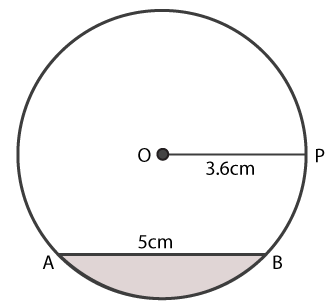(i) OP is the radius of the circle i.e OP = 3.6 cm

AB is the chord of the circle i.e AB = 5 cm

(ii) Minor segment of the circle is shaded in the given circle

7. Mark two points A and B, 4 cm a part. Draw a circle passing through B and with A as a centre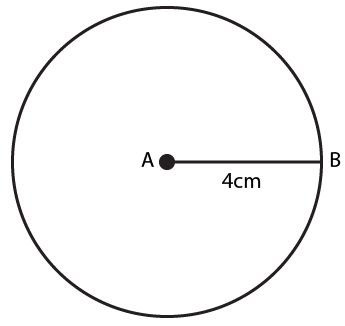Solution:

In the given figure A is the centre of the circle and

AB is the radius of the circle i.e AB = 4 cm

8. Draw a line AB = 8.4 cm. Now draw a circle with AB as diameter. Mark a point C on the circumference of the circle. Measure angle ACB.

Solution: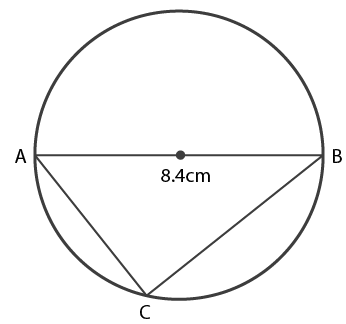By measurement we get,

∠ACB = 900